# Important 3 Marks Questions for CBSE 12th Chemistry

Class 12 chemistry important 3 mark questions with answers are provided here. These important questions are based on CBSE board curriculum and correspond to the most recent Class 12 chemistry syllabus. By practicing these Class 12 important 3 mark questions, students will be able to quickly review all of the ideas covered in the chapter and prepare for the Class 12 Annual examinations as well as other entrance exams such as NEET and JEE.

Download Class 12 Chemistry Important 3 Mark Questions with Answers PDF by clicking on the button below.

## Boards End Game – Chemistry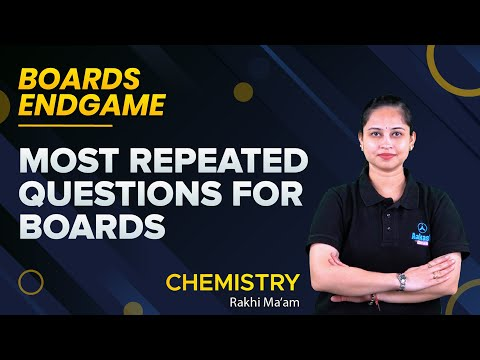## Class 12 Chemistry Important 3 Mark Questions with Answers

Question 1:

The partial pressure of ethane over a saturated solution containing 6.56 x 10-2 of ethane is 1 bar. If the solution were to contain 5.0 x 10-2 g of ethane, what will be the partial pressure of the gas.

For a gas that has a very high solubility, we may assume that the partial pressure of the gas is directly proportional to its mass.

Here, P ∝ m

$$\begin{array}{l}\frac{P_{2}}{P_{1}}=\frac{m_{2}}{m_{1}}\end{array}$$
$$\begin{array}{l}P_{2}=P_{1}\times \frac{m_{2}}{m_{1}}\end{array}$$
$$\begin{array}{l}P_{2}=1\times \frac{0.05}{0.0656}=0.762 bar\end{array}$$

Question 2:

(a)What are fuel cells? Explain the electrode reactions involved in working of H2 – O2 fuel cell.

(b)Represent the galvanic cell in which the reaction Zn + Cu2+ → Zn2+ + Cu , take place.

(a) Fuel cells are cells that convert the chemical energy of a fuel directly into electrical energy. The electrode reactions are as follows:

Anode:

$$\begin{array}{l}\left [H_{2}+2OH^{-}\to 2H_{2}O+2e^{-} \right ]\times 2 \end{array}$$

Cathode:

$$\begin{array}{l}O_{2}+H_{2}O+4e^{-}\to 4OH^{-}\end{array}$$

Net reaction:

$$\begin{array}{l}2H_{2}+O_{2}\to 2H_{2}O\end{array}$$

(b) Galvanic cell may be represented as –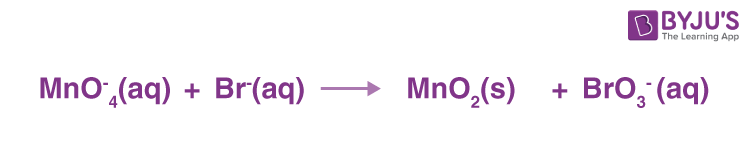Question 3:

(a)Based on the nature of intermolecular forces, classify the following solids: Silicon carbide, Argon

(b)ZnO turns yellow on heating. Why?

(c)What is meant by groups 12-16 compounds? Give an example.

(a) Based on the nature of intermolecular forces:

Silicon carbide can be classified as Covalent solid

Argon can be classified as Molecular solid (non-polar).

(b) Zn2+ ions occupy the interstitial sites in ZnO, and electrons are trapped in the interstitial sites for neutralisation. Crystals with these types of flaws are usually coloured. Zinc oxide is white in colour, but it turns yellow when heated because it loses oxygen reversibly at high temperatures.

(c) Compounds formed by combining elements from groups 12 and 16 are referred to as 12-16 group compounds. ZnS, CdS, CdSe, and HgTe are a few examples.

Compounds formed by combining groups 12 and 16 behave in the same way as semiconductors.

Question 4:

(a)The cell in which the following reaction occurs: 2Fe3+ (aq) + 2I(aq) → 2Fe2+ (aq) + I2(s) has cell E°cell= 0.236 V at 298 K. Calculate the standard Gibbs energy of the cell reaction. (Given : 1F = 96,500 C mol-1)

(b)How many electrons flow through a metallic wire if a current of 0·5 A is passed for 2 hours? (Given: 1F = 96,500 C mol-1)

(a) ΔG = nFE° = – 2 ✖ 96500 ✖ 0.236 = -45.548 kJ

$$\begin{array}{l}log K=\frac{nFE^{\circ }}{2.303RT}=\frac{2\times 0.236}{0.0591}=7.986\end{array}$$
$$\begin{array}{l}K=10^{7.986}=96827785.6=9.6\times 10^{7}\end{array}$$

(b) Charge passed:

q= IT = 0.5 A ✖ 2 ✖ 60 ✖ 60 = 3600 C

F = 3600/96500

Number of electrons passed:

$$\begin{array}{l}N_{e}=\frac{3600}{96500}\times 6.02\times 10^{23}= 2.24\times 10^{22}electrons\end{array}$$

Question 5:

(a)What type of isomerism is shown by the complex: [Co(NH3)5(SCN)]2+?

(b)Why is [NiCl4]2– paramagnetic while [Ni(CN)4]2– is diamagnetic? (Atomic number of Ni = 28)

(c)Why are low spin tetrahedral complexes rarely observed?

(a) Linkage isomerism is shown by the complex [Co(NH3)5(SCN)]2+.

(b) This is due to the different nature of the ligands. Cl is a weak field ligand, so it does not pair unpaired 3d electrons. As a result, [NiCl4]2– is paramagnetic.

CN, on the other hand, is a strong field ligand. As a result, it leads to the pairing of unpaired 3d electrons. Since there are no unpaired electrons in this case, [Ni(CN)4]2– is diamagnetic.

(c) When a strong field ligand bonds with a metal or ion, low spin complexes form. The strong field ligand causes electron pairing, which leaves the last d-orbital empty, preventing tetrahedral formation. In addition, the d-orbital splitting is small in comparison to the octahedral splitting. As a result, the orbital splitting energy is insufficient to force pairing. As a result, low spin configurations in tetrahedral complexes are rarely observed.

Question 6:

Write one difference in each of the following :

(a) Multimolecular colloid and Associated colloid

(b) Coagulation and Peptization

(c) Homogeneous catalysis and Heterogeneous catalysis

(a) Multimolecular colloids are made up of aggregates of many atoms or smaller molecules. It has a wavelength range of (1-1000)nm.
Associated colloids: They act as strong electrolytes at low concentrations and form aggregates or micelles at high concentrations.

(b) Peptization is the process of dispersing precipitate into colloidal particles, whereas coagulation is the process by which colloidal particles join to form large-sized particles.

(c) The catalyst in a heterogeneous reaction is in a different phase than the reactants. The catalyst is in the same phase as the reactants in a homogeneous reaction.

Question 7:

(a) Write the dispersed phase and dispersion medium of milk.

(b) Write one similarity between physisorption and chemisorption.

(c) Write the chemical method by which Fe(OH)3 sol is prepared from FeCl3.

(a) The dispersed phase and dispersion medium of milk both are liquid (Emulsion).

(b) Physisorption and chemisorption are similar in that they are both surface phenomena.

(c)

$$\begin{array}{l}FeCl_{3}+3H_{2}O\xrightarrow[]{Hydrolysis}Fe\left ( OH \right )_{2}+3HCl\end{array}$$

Question 8:

A first-order reaction takes 20 minutes for 25% decomposition. Calculate the time when 75% of the reaction will be completed.

(Given : log 2 = 0·3010, log 3 = 0·4771, log 4 = 0·6021)

A first-order reaction takes 20 minutes for 25% decomposition.

$$\begin{array}{l}k=\frac{2.303}{t} log\frac{a}{\left ( a-x \right )}\end{array}$$
$$\begin{array}{l}k=\frac{2.303}{20min} log\frac{a}{\left ( a-\left ( 0.25a \right ) \right )}\end{array}$$
$$\begin{array}{l}k=\frac{2.303}{20min} log\frac{a}{0.75}\end{array}$$

k=0.0144/min

When 75% of the reaction will be completed.

$$\begin{array}{l}k=\frac{2.303}{t} log\frac{a}{\left ( a-x \right )}\end{array}$$
$$\begin{array}{l}k=\frac{2.303}{0.0414/min} log\frac{a}{\left ( a-0.75a \right )}\end{array}$$
$$\begin{array}{l}k=\frac{2.303}{0.0414/min} log4\end{array}$$
$$\begin{array}{l}k=\frac{2.303}{0.0414/min}\times 0.6021\end{array}$$

t = 96.4 min.

Question 9:

The following compounds are given to you : 2-Bromopentane, 2-Bromo-2-methylbutane, 1-Bromopentane

(a) Write the compound which is most reactive towards SN2 reaction.

(b) Write the compound which is optically active.

(c) Write the compound which is most reactive towards the -elimination reaction.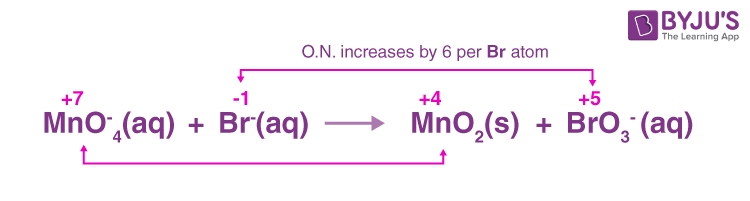(a) 1-bromopentane will be the most reactive to SN2 because it is the least hindered.

For the SN2 reaction, primary alkyl halides are the most reactive.

(b) 2-Bromopentane has chiral carbon in it. So this compound is optically active.

(c) 2-Bromo-2-methyl pentane will give a stable elimination product.

Question 10:

Write the principle of the following :

(a) Zone refining

(b) Froth floatation process

(c) Chromatography

(a) Based on the principle that impurities are more soluble in a molten state than in a solid-state.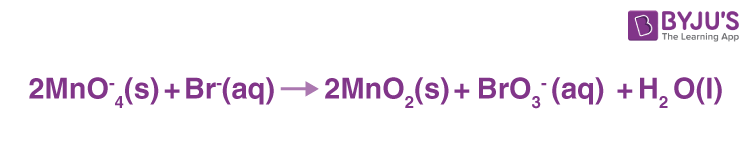(b) Based on the fact that oil wets mineral particles while water wets gangue particles.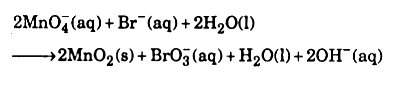(c) On the basis of the principle that different components of a mixture are adsorbed differently on an adsorbent.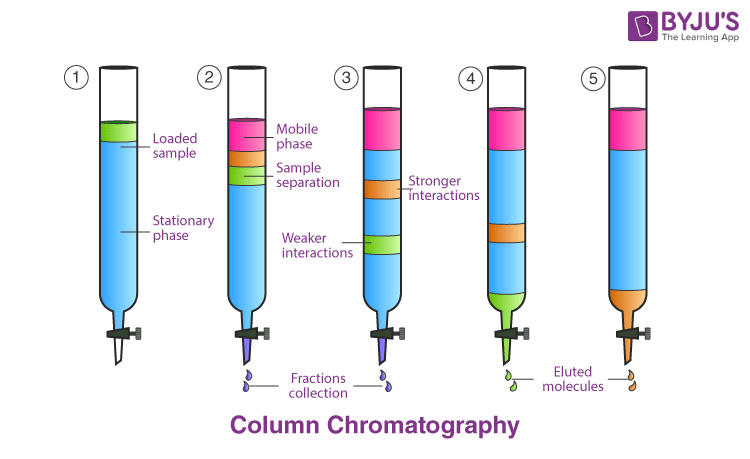Question 11:

Write the structures of the monomers used for getting the following polymers :

(a) Nylon-6,6

(b) Melamine-formaldehyde polymer

(c) Buna-S

(a) The monomer of Nylon-6, 6 is Hexamethylenediamine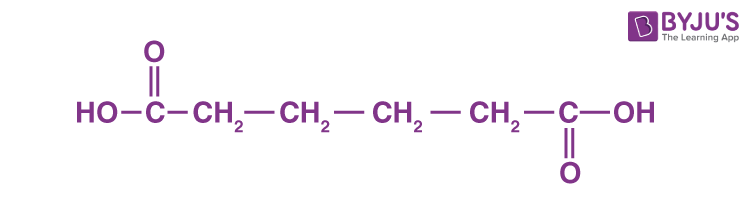(b)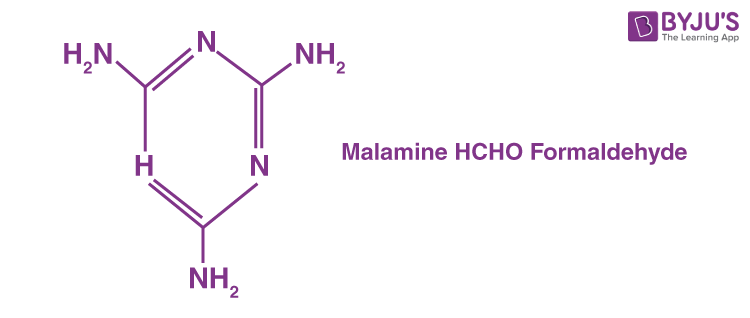(c)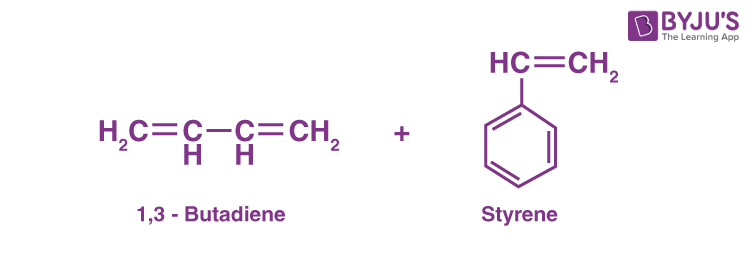Question 12:

Define the following :

(a) Anionic detergents

(b) Limited spectrum antibiotics

(c) Antiseptics

(a) Anionic detergents are sodium salts of sulfonated long-chain alcohol or hydrocarbons/alkylbenzene sulphonate or detergents with an anionic component that performs cleaning.

(b) Antibiotics with a narrow spectrum of action are those that are only effective against a single organism or disease.

(c) Antiseptics are chemicals that either kill or prevent microbe growth on living tissue.

Question 13:

Give reasons for the following :

(a) Red phosphorus is less reactive than white phosphorus.

(b) Electron gain enthalpies of halogens are largely negative.

(c) N2O5 is more acidic than N2O3.

(a) White phosphorus is made up of discrete P4 tetrahedrons that are subjected to extremely high angular strain due to the angles being 60°. Red phosphorus is a polymer of P4 tetrahedra with a much lower angular strain than white phosphorus. Because of the high angular strain, white phosphorus becomes unstable and highly reactive.

(b) In their respective periods, halogens are the smallest in size, resulting in a high effective nuclear charge. To achieve noble gas electronic configuration, they accept one electron. As a result, they have a negative electron gain enthalpy.

(c) The oxidation state of N in N2O5 is +5. The oxidation state of N in N2O3 is +3. Since N2O5 has a higher oxidation state, it is more acidic.

Question 14:

Give reasons for the following :

(a) Acetylation of aniline reduces its activation effect.

(b) CH3NH2 is more basic than C6H5NH2

(c) Although -NH2 is o/p directing group, aniline on nitration gives a significant amount of m-nitroaniline.

(a) Since resonance decreases electron density on nitrogen, acetylation of aniline reduces its activation effect because the formed acetoamido group is less activating than the amino group.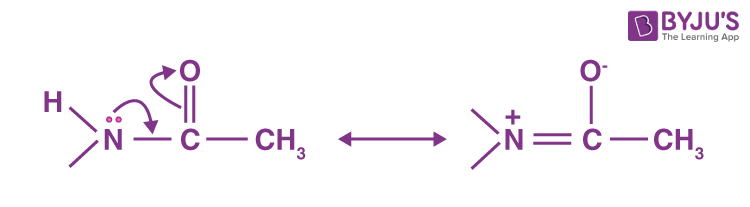(b) CH3NH2 is more basic than CH5NH2 because the electron density on nitrogen decreases and the anilinium ion formed is more reasonably stable.

(c) Nitration takes place in an acidic medium. Aniline is protonated in a strongly acidic medium to produce anilinium ion (which is meta-directing). As a result, aniline on nitration yields a significant amount of m-nitroaniline.

Question 15:

Define the following terms given an example of each

(i) Associated colloids

(ii) Lyophilic solution

(i) Associated Colloids-Some colloids behave as normal electrolytes at low concentrations but exhibit colloidal behaviour at higher concentrations due to aggregate formation. Associated colloids, for

(ii) Lyophilic sol is the substance that, when mixed with a suitable liquid as the dispersion medium, directly forms a colloidal sol. They are reversible and quite stable in nature and do not readily precipitate or coagulate. However, very large amounts of electrolytes can cause particles to precipitate.

(iii) Adsorption is the adhesion of atoms, ions, or molecules to a surface from a gas, liquid, or dissolved solid. This process forms an adsorbate film on the adsorbent’s surface.

Question 16:

Calculate the emf of the following cell at 25ºC Ag (s) | Ag+ (10-3 M) || Cu2+(10-1 M) | Cu (s) Given E°cell = +0.46 V and log10n = n

Cathode: Cu2+ + 2e → Cu

Anode: 2Ag → 2Ag+ + 2e

Cell Reaction: Cu2+ + 2Ag → Cu + 2Ag+

cell = +0.46 V

$$\begin{array}{l}E_{cell}^{\circ }= 0.46V – \frac{0.0591}{2} log \frac{\left [ Ag^{+} \right ]^{2}}{\left [ Cu^{2+} \right ]}\end{array}$$
$$\begin{array}{l}E_{cell}^{\circ }= 0.46V – \frac{0.0591}{2} log \frac{\left [ 10^{-3} \right ]^{2}}{\left [ 10^{-1} \right ]}\end{array}$$
$$\begin{array}{l}E_{cell}^{\circ }= +0.61V\end{array}$$

Question 17:

Shanti, a domestic helper of Mrs. Anuradha, fainted while mopping the floor. Mrs. Anuradha immediately took her to the nearby hospital where she was diagnosed to be severely ‘anaemic.’ The doctor prescribed an iron-rich diet and multivitamins supplement to her. Mrs. Anuradha supported her financially to get the medicines. After a month, Shanti was diagnosed to be normal.

(i) What values are displayed by Mrs. Anuradha?

(ii) Name the vitamin whose deficiency causes ‘pernicious anaemia’.

(iii) Give an example of a water-soluble vitamin.

(i) Mrs. Anuradha exemplifies high moral and humanitarian values.

(ii) The loss of stomach cells that produce intrinsic factors is the most common cause of pernicious anaemia. Intrinsic factor aids in the absorption of vitamin B12 in the intestine. The destruction of parietal cells by the body’s own immune system could be the cause of their loss.

(iii) Thiamin, a member of the vitamin B-complex group (vitamin B1) is an example of a water-soluble vitamin.

Question 18:

The rate of a reaction becomes four times when the temperature changes from 293 K to 313 K. Calculate the energy of activation (Ea) of the reaction assuming that it does not change with temperature. [R = 8.314 J K mole-1, log 4 = 0.6021]

$$\begin{array}{l}log\frac{k_{2}}{k_{1}}=\frac{E_{a}}{2.303R}\left ( \frac{1}{T_{1}}-\frac{1}{T_{2}} \right )\end{array}$$
$$\begin{array}{l}log4=\frac{E_{a}}{2.303\times 8.314}\left ( \frac{1}{T_{1}}-\frac{1}{T_{2}} \right )\end{array}$$
$$\begin{array}{l}{E_{a}}=0.6021\times 2.303\times 8.314\times 293\times \frac{313}{20}\end{array}$$
$$\begin{array}{l}{E_{a}}=52.863 KJ\end{array}$$

Question 19:

For the complex [NiCl4]-2, write

(i) The IUPAC name

(ii) The hybridization type

(iii) The shape of the complex (Atomic no. of Ni = 28)

(i) The IUPAC name of the complex is tetrachloronickelate (II) ion

(ii) One s and three p orbitals are hybridised to form four equivalent tetrahedral orbitals. Therefore, the hybridization is sp3.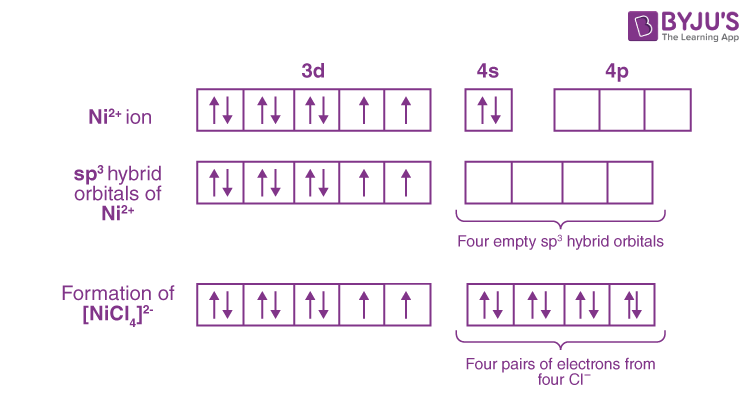(iii) The shape of the complex is tetrahedral.Question 20:

(i) What is meant by crystal field splitting energy?

On the basis of crystal field theory, write the electronic configuration of d4 in terms of t2g and eg in an octahedral field when-

(ii) ∆0 > P

(iii) ∆0 < P

(i) Crystal field splitting energy is defined as the difference in energy between two sets of degenerated orbitals after crystal field splitting.

(ii) Crystal field splitting energy is defined as the difference in energy between two sets of degenerated orbitals after crystal field splitting. If ∆0 > P, the fourth electron has a better chance of occupying a t2g orbital with the configuration [t2g]4 [eg]0. Strong field ligands form low spin complexes and are known as strong field ligands.

(iii) If ∆0 < P, strong-field ligands form low spin complexes and are known as strong field ligands. The configuration, in this case, is [t2g]3 [eg]1.

Question 21:

Give reasons for the following

(i) Ethyl iodide undergoes SN2 reaction faster than ethyl bromide.

(ii) (±) 2-Butanol is optically inactive.

(iii) C-X bond length in halobenzene is smaller than C-X bond length in CH3 – X.

(i) Since size increases as we move down the periodic table, ethyl iodide undergoes the SN2 reaction faster than ethyl bromide. As the size of the group grows, basicity decreases and the ability of the leaving group to leave grows. Because iodine is a fast leaving group, it undergoes the SN2 reaction faster than ethyl bromide.

(ii) (±) 2-butanol is a racemic mixture that is optically inactive due to external compensation.

(iii) Halobenzene has a lower bond length value than CH3X due to resonance.

Question 22:

(i) What class of drug is Ranitidine?

(ii) If the water contains dissolved Ca2+ ions, out of soaps and synthetic detergents, which will you use for cleaning clothes?

(iii) Which of the following is an antiseptic? 0.2% phenol, 1% phenol

(i) Ranitidine belongs to antihistamines. Therefore, it belongs to the class of drugs known as antacids.

(ii) Ca2+ hardens water. As a result, soap cannot be used because it precipitates in hard water. A synthetic detergent, on the other hand, does not precipitate in hard water because its calcium salt is also soluble in water. As a result, synthetic detergents can be used to wash clothes in hard water.

(iii) 0.2% phenol can be used as an antiseptic whereas 1% phenol can be used as a disinfectant.

Question 23:

Given reasons for the following :

(i) Oxygen is gas but sulphur is solid.

(ii) O3 acts as a powerful oxidizing agent.

(iii) BiH3 is the strongest reducing agent amongst all the hydrides of group 15 elements.

(i) Because of its small size and high electronegativity, oxygen forms a pπ- pπ double bond with another oxygen atom (O = O) to form O2 molecules. At room temperature, the intermolecular forces of attraction between O2 molecules are weak van der Waals forces, so oxygen is a diatomic gas. However, because of its larger size, sulphur does not form p-p double bonds and thus does not form diatomic molecules. It is found as octa atomic molecules ( S8) with a puckered ring structure. The attraction forces that hold, (S8) molecules together are much stronger. As a result, sulphur is solid.

(ii) The compound O3 is thermodynamically unstable. When heated, it easily decomposes into dioxygen and nascent oxygen. Since nascent oxygen is highly reactive, O3 acts as a powerful oxidising agent.

(iii) BiH3 is the least stable hydride of group 15 elements due to the largest size of Bi, resulting in a very weak Bi – H bond. As a result, it has the greatest proclivity to liberate hydrogen. As a result, it is the most powerful reducing agent among the hydrides of group 15 elements.

Question 24:

(i) Write the IUPAC name of the complex [Cr(NH3)4Cl2]Cl.

(ii) What type of isomerism is exhibited by the complex [Co(en)3]3+? (en = ethane-1,2-diamine)

(iii.) Why is [NiCl4 ]2– paramagnetic but [Ni(CO)4 ] is diamagnetic? (Cr=24, Co=27, Ni=28)

(i) The IUPAC name of the complex [Cr(NH3)4Cl2]Cl is Tetraamminedichlorochromium(III) chloride.

(ii) The complex [Co(en)3]3+ exhibits optical isomerism.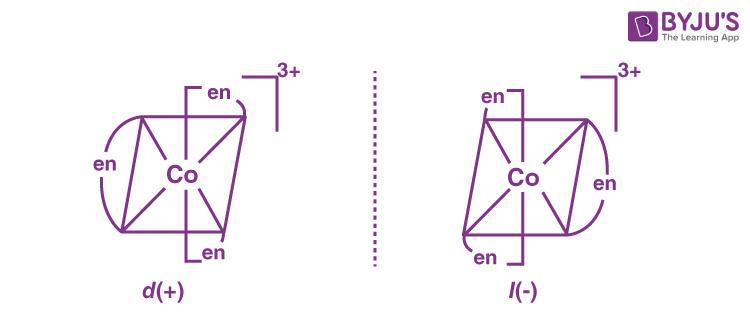(iii) The oxidation state of Ni in [NiCl4 ]2– is +2. Chloride is a weak field ligand that does not cause electron pairing up, which violates Hund’s rule of maximum multiplicity. As a result, two unpaired electrons are present in the valence d-orbitals of Ni, giving the complex a paramagnetic character. Carbonyl, on the other hand, is a strong field ligand that causes electron pairing up, which goes against Hund’s rule of maximum multiplicity. As a result, there are no unpaired electrons, and the complex is diamagnetic.

Question 25:

Account for the following :

(i) Primary amines (R-NH2) have a higher boiling point than tertiary amines (R3N).

(ii) Aniline does not undergo Friedel-Crafts reaction.

(iii) (CH3)2NH is more basic than (CH3)3N in an aqueous solution.

(i) Primary amines participate in intermolecular hydrogen bonding, whereas tertiary amines lack available hydrogen atoms and thus do not participate in intermolecular hydrogen bonding. As a result, primary amines (R-NH2) have a higher boiling point than secondary amines (R3N).

(ii) In the presence of a Lewis acid, such as AlCl3, a Friedel-Crafts reaction takes place. Since aniline is basic in nature, it reacts with AlCl3 to form a salt.

(iii) (CH3)2NH is hydrated to a greater extent than (CH3,)3NH. Because of steric hindrance, the extent of hydration decreases as the number of methyl groups increases. The greater the extent of hydration, the greater the ion stability and the basic strength of amine.

Question 26:

On the occasion of World Health Day, Dr. Satpal organized a ‘health camp’ for the poor farmers living in a nearby village. After check-up, he was shocked to see that most of the farmers suffered from cancer due to regular exposure to pesticides and many were diabetic. They distributed free medicines to them. Dr. Satpal immediately reported the matter to the National Human Rights Commission (NHRC). On the suggestions of NHRC, the government decided to provide medical care, and financial assistance and set up of super-speciality hospitals for treatment and prevention of the deadly disease in the affected villages all over India.

(i) Write the values shown by

(a) Dr. Satpal

(b) NHRC

(ii) What type of analgesics are chiefly used for the relief of pains of terminal cancer?

(iii) Give an example of an artificial sweeteners that could have been recommended to diabetic patients.

(i) (a) Dr. Satpal feels concerned for society. He is pained to see the people suffering from cancer and diabetes. He shows courage to report the matter to NHRC so that the suffering people can be helped in a proper way. Thus, he shows values of kind-heartedness, social concern, helpfulness and fighting spirit.

(b) NHRC shows values of social responsibility, promptness to provide relief to affected people, and above all concern for people suffering from deadly cancer and diabetes.

(ii) Narcotic analgesics.

(iii.) Saccharin is an artificial sweetener that could be recommended to diabetic patients.

Question 27:

Define the following terms :

(i) Nucleotide

(ii) Anomers

(iii) Essential amino acids

(i) Nucleotides are organic molecules that function as monomers, or subunits, in nucleic acids such as DNA (deoxyribonucleic acid) and RNA (ribonucleic acid). Nucleotides are the building blocks of nucleic acids, consisting of a nitrogenous base, a five-carbon sugar (ribose or deoxyribose), and at least one phosphate group. A nucleotide is formed by combining a nucleoside with a phosphate group.

(ii) In carbohydrate chemistry, an anomer is a type of stereoisomer and epimer. An anomer is a cyclic saccharide and an epimer that differs in configuration, specifically at the hemiacetal/acetal carbon, also known as the anomeric carbon. The carbon derived from the carbonyl carbon (the ketone or aldehyde functional group) of the open-chain form of the carbohydrate molecule is known as anomeric carbon.

(iii) An essential amino acid, also known as an indispensable amino acid, is an amino acid that cannot be synthesised by the organism and must therefore be obtained from the diet.

Question 28:

(i) Calculate ∆rGº for the reaction

Mg(s) + Cu2+ (aq) → Mg2+ (aq) + Cu(s)

Given : Eºcell = + 2.71 V, 1 F = 96500 C mol–1.

(ii) Name the type of cell that was used in Apollo space program for providing electrical power.

(i) For the cell reaction,

Mg(s) + Cu2+ (aq) → Mg2+ (aq) + Cu(s); Eºcell = + 2.71 V

The change in standard Gibb’s free energy can be given by:

∆rGº = -nFEºcell = – 2 ✖ 96500 ✖ 2.71= –523030 J mol–1

(ii) The fuel cell, which uses the reaction of hydrogen with oxygen to form water, was used to provide electrical power in the Apollo space programme.

Question 29:

What are emulsions? What are their different types? Give one example of each type.

An emulsion is a mixture of two or more normally immiscible liquids (non-mixable or unblendable). It means that the dispersed phase and dispersion medium in an emulsion are both liquids. There is a continuous phase (dispersion medium) in every emulsion that suspends the droplets of the other element, which is known as the dispersed phase.
Emulsions are classified into two types: oil in water and water in oil.

• Oil-in-water emulsions (O/W): A milk emulsion is an example of an oil-in-water emulsion. Liquid fat globules are dispersed in water in milk. Other examples include vanishing cream and so on.
• Water-in-oil emulsion (W/O): These emulsions are commonly found in butter and cold cream. Other examples include cod liver oil.

Question 30:

Given reasons for the following :

(i) (CH3)3P = O exists but (CH3)3N = O does not.

(ii) Oxygen has less electron enthalpy with a negative sign than sulphur.

(iii) H3PO2 is a stronger reducing agent than H3PO3.

(i) Due to the lack of low lying vacant d- orbitals, the N atom cannot expand its covalency beyond four, whereas the P atom has low lying vacant d- orbitals. As a result (CH3)3P = O exists but (CH3)3N = O does not.

(ii) The incoming electron is not easily accommodated due to the oxygen atom’s small size and compact nature. As a result, oxygen has a lower negative electron gain enthalpy than sulphur.

(iii) The greater the number of element-hydrogen (EH) bonds present in a compound, the more reducing the compound is. H3PO2 has two P-H bonds, whereas H3PO3 only has one PH bond. Therefore, H3PO2 is a stronger reducing agent than H3PO3.

Question 31:

(i) Name the method used for the refining of titanium

(ii) What is the role of Zn in the extraction of silver?

(iii) Reduction of metal oxide to metal becomes easier if the metal obtained is in the liquid state. Why?

(i) Van Arkel method is used for refining Zirconium. By heating metal in an evacuated vessel with iodine, oxygen and nitrogen present as impurities are removed. The metal iodide volatilizes and decomposes on a tungsten filament heated to approximately 1800 K. On the filament, pure metal is deposited.

Zone refining is a technique for purifying materials used as semiconductors in electronic devices.

(ii) During silver extraction, finely powdered silver ore is treated with dilute KCN solution to form a silver cyano complex. This complex dissolves in water.

Ag2S + 4NaCN ⇌ 2Na[Ag(CN)2] + Na2S

The solution is then treated with zinc. Silver is displaced from the complex by more electropositive zinc.

2Na[Ag(CN)2] + Zn → Na2[Zn(CN)4] +2Ag↓

(iii) The temperature of the substance when it is liquid is higher. According to the Ellingham diagram, the value of G for the formation of metal oxides increases with increasing temperature. If the Gibbs energy is negative, the metal oxide can be reduced to metal. As a result, reducing the metal oxide to metal is easier if the metal is obtained in liquid form.

Question 32:

1. E° value for the Mn3+/Mn2+ couple is positive (+1.5 V) whereas that of Cr3+/Cr2+ is negative (– 0.4 V). Why?
2. Transition metals form coloured compounds. Why?
3. Complete the following equation :
$$\begin{array}{l}2MnO_{4}^{-}+16H^{+}+5C_{2}O_{4}^{2-}\to \end{array}$$

(i) Since Mn3+ has a 3d4 outer electronic configuration and Mn2+ has a 3d5 outer electronic configuration. As a result, the conversion of Mn3+ to Mn2+ will be a favourable reaction because 3d5 is a very stable configuration due to its half-filled nature. As a result, the E° value for the Mn3+/ Mn2+ couple is positive.

The outer electronic configuration of Cr3+ is 3d3 (half-filled d orbitals) and extra stability is attained by Cr3+ than Cr2+. Hence, Cr3+ → Cr2+ is less feasible, and the resultant configurations are not stable and thus have a lower E° value.

(ii) In general, transition metal ions have one or more unpaired electrons. When visible light strikes a transition metal compound or ion, the unpaired electrons in the lower energy d-orbital are promoted to higher energy d-orbitals, a process is known as the d-d transition. Because the energy involved in the d-d transition is quantized, only a specific wavelength is absorbed, while the remaining wavelengths in the visible region are transmitted. As a result, transmitted light contains some complementary colour to the absorbed colour.

(iii)

$$\begin{array}{l}2MnO_{4}^{-}+16H^{+}+5C_{2}O_{4}^{2-}\to 10CO_{2}+2Mn^{2+}+8H_{2}O\end{array}$$

Question 33:

(i) What type of isomerism is shown by [CO(NH3)5ONO]Cl2 ?

(ii) On the basis of crystal field theory, write the electronic configuration for d4 ion if ∆0< P

(iii) Write the hybridization and shape of [Fe(CN)6]3– (Atomic number of Fe = 26)

(i) The complex [Co(NH3)5ONO]Cl shows Linkage isomerism and the linkage isomer is [Co(NH3)NO2]Cl.

(ii) If ∆0 < P, strong-field ligands form low spin complexes and are known as strong field ligands. The configuration, in this case, is [t2g]3 [eg]1

(iii) CN is a powerful field ligand and uses inner 3d-orbitals to form a low spin complex. As there are 6 ligands, it has octahedral geometry. The Cyano group being a strong field ligand will create a low spin complex using inner 3d orbitals. As there are six ligands present, it will form octahedral geometry. Therefore, the hybridisation present is d2sp3.

Question 34:

Write the names and structures of the monomers of the following polymers :

(i) Nylon-6,6

(ii) Bakelite

(iii) Polystyrene

(i) It is a polymer formed by the condensation of adipic acid and hexamethylenediamine. The number 6 denotes the number of carbon atoms in each monomer.

Hexamethylenediamine structure is H2N(CH2)6NH2 and the structure of adipic acid is HOOC – (CH2)4 COOH.

(ii) Bakelite is a condensation polymer of phenol C6H5OH and formaldehyde HCHO.

(iii) Polystyrene is a synthetic aromatic polymer made from the monomer styrene.Question 35:

(i) Which one of the following is a disaccharide: Starch, maltose, fructose, glucose

(ii) What is the difference between acidic amino acid and basic amino acid?

(iii) Write the name of the linkage joining two nucleotides.

(i) Maltose is a disaccharide, as it contains α−D glucose units.

(ii) Amino acids, which contain an amino group (NH2) and a carboxyl group (-COOH), are the basic building blocks of proteins. The acidic amino acids have an extra carboxyl group in their structure, whereas the basic amino acids have an extra amino group in their structure.

(iii) Amino acids can be combined to form amides known as peptides. The peptide bond refers to the – CO-NH-bond or linkage. A peptide bond is formed by the loss of water from a 2-amino acid.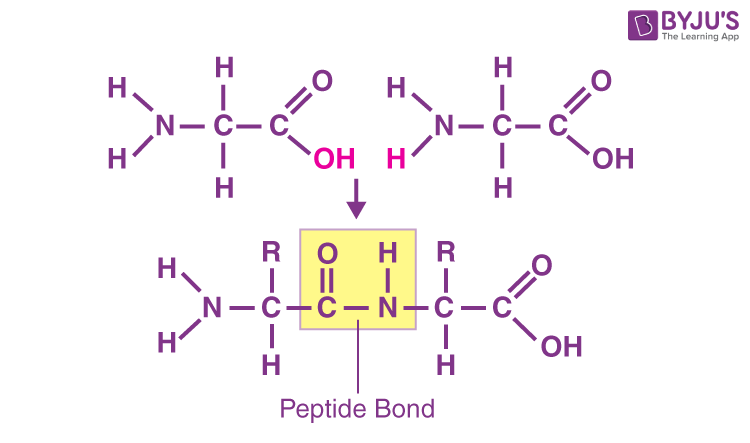Question 36:

The Vapour pressure of water at 20ºC is 17.5 mm Hg. Calculate the vapour pressure of water at 20ºC when 15 g of glucose (Molar mass = 180 g mol-1) is dissolved in 150 g of water.

According to Raoult’s law:

$$\begin{array}{l}\frac{P_{A}-P_{S}}{P_{S}}=\frac{n_{b}}{n_{a}}=\frac{W_{B}}{M_{B}}\times \frac{M_{A}}{W_{A}}\end{array}$$
$$\begin{array}{l}\frac{17.5mm-P_{S}}{P_{S}}=\frac{15g}{180 gmol^{-1}}\times \frac{18g mol^{-1}}{150g}= 0.01\end{array}$$
$$\begin{array}{l}\frac{17.5mm}{P_{S}}-1=0.01\end{array}$$
OR
$$\begin{array}{l}\frac{17.5mm}{P_{S}}=1.01\end{array}$$
$$\begin{array}{l}{P_{S}}=\frac{17.5mm}{1.01}=17.326 mm\end{array}$$

Question 37:

Examine the given defective crystal :

X+ Y– X+ Y– X+

Y– X+ Y– X+ Y–

X+ Y– X+ e– X+

Y– X+ Y– X+ Y–

(i) Is the above defect stoichiometric or non-stoichiometric?

(ii) Write the term used for the electron occupied site.

(iii) Give an example of the compound which shows this type of defect.

(i) The above defect is Non-stoichiometric.

(ii) F-centre.

(iii) When LiCl is heated with lithium vapours, it shows this type of defect.

Question 38:

How do you convert the following :

(i) Prop-1-ene to Propan-2-ol

(ii) Bromobenzene to 2-bromoacetophenone

(iii) 2-bromobutane to But-2-ene

(i) Propan–2–ol is formed when propene reacts with water in the presence of an acid as a catalyst.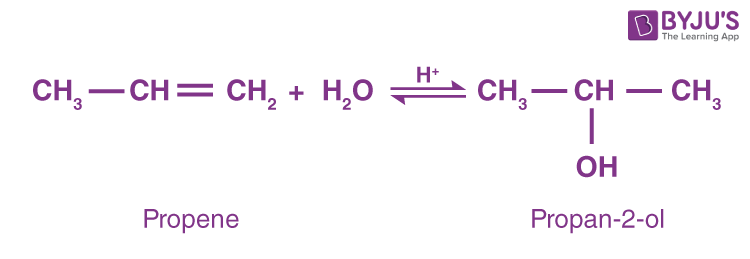(ii) Friedel-crafts acylation converts bromobenzene to 2-bromoacetophenone. Bromobenzene undergoes acyl chloride treatment in the presence of FeCl3/AlCl3 to produce 4-bromoacetophenone (major product) and 2-bromoacetophenone.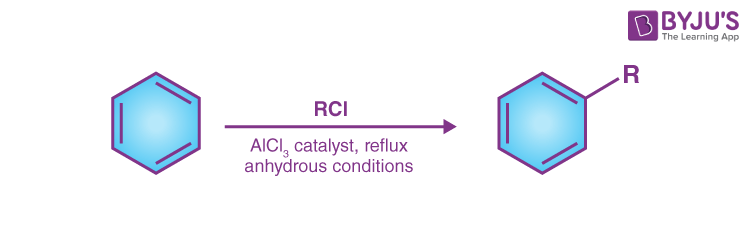(iii) 2-Bromobutane on heating with alc. KOH undergoes dehydrohalogenation to give but-2-ene as the major product.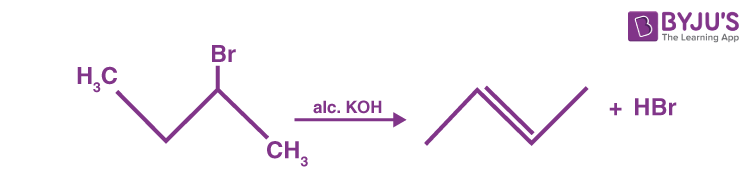Question 39:

Give reasons for the following :

(i) p-nitrophenol is more acidic than p-methyl phenol.

(ii) Bond length of C – O bond in phenol is shorter than that in methanol.

(iii) (CH3)3C – Br on reaction with sodium methoxide (Na+OCH3) gives alkene as the main product and not an ether.

(i) p-nitrophenol is more acidic than p-methyl phenol. This is due to the fact that p-nitrophenol has an electron-withdrawing group, which is more effective. While p-methylphenol has a methoxy group which has lesser electrons withdrawing group.

(ii) The bond length of the C-O bond in phenol is shorter than that of the CH3OH bond due to the following facts:

• Conjugation of an unshared electron pair over oxygen with an aromatic ring in phenol results in a partial double bond character in the carbon-oxygen bond.
• In phenol, oxygen is attached to an sp2 hybridised carbon atom, whereas oxygen is attached to an sp3 hybridized carbon atom in methanol.

(iii) Whenever an alkyl halide is made to react with phenoxide or alkoxide we get ether. This does not happen when (CH3)3C – Br reacts with sodium methoxide (Na+OCH3) instead an alkene is produced.

(CH3)3C – Br + (Na+OCH3) → (CH3)3C = CH2

This happens because whenever secondary or tertiary alkyl halides are made to undergo Williamson ether synthesis they will always show elimination instead of substitution.

Question 40:

Calculate E°cell and ∆rG° for the following reaction at 25ºC :

A2+ + B+ → A3+ + B

Given : KC = 1010 and F = 96500 C mol–1

$$\begin{array}{l}\Delta G= -nFE^{\circ }= -2.303 RT log K_{c}\end{array}$$
$$\begin{array}{l}E^{\circ }= \frac{2.303 RT}{nF}log K_{c}\end{array}$$

At T = 298 K,

$$\begin{array}{l}E^{\circ }= \frac{0.059}{1}log 10^{10}\end{array}$$

E° = 0.59 V

ΔG° = -nFE° = -1 ✖ 96500 ✖ 0.59 = 56935 J = 56.935 KJ.

Question 41:

Adsorption is the accumulation of molecular species at the surface rather than in the bulk of a solid or liquid. Water vapour, for example, is adsorbed by silica gel.

When gas gets absorbed on the surface of a solid, its entropy decreases, causing S to become -ve. Since adsorption is a spontaneous process, ΔG(=ΔH−TΔS) must be negative. But since −TΔS is positive, G can only be negative if H has a sufficiently high -ve value. As a result, adsorption is exothermic in nature.

Based on the nature of the forces between adsorbate and adsorbent, there are two types of adsorption.

• Physical adsorption occurs when gas accumulates on the surface of a solid due to weak van der Waal forces.
• Chemical adsorption occurs when gas molecules or atoms are chemically bonded to the surface of a solid.

Question 42. What happens when

(i) Ethyl chloride is treated with NaI in the presence of acetone,

(ii) Chlorobenzene is treated with Na metal in the presence of dry ether,

(iii) Methyl chloride is treated with KNO2?

(i) Ethyl iodide is formed as a result of halogen exchange, which is known as the Finkelstein reaction.

$$\begin{array}{l}CH_{3}Cl + NaI\xrightarrow[]{Acetone}C_{2}H_{5}I +NaCl\end{array}$$

(ii) When Chlorobenzene is treated with Na metal in the presence of dry ether Fittig reaction takes place which results in the formation of diphenyl.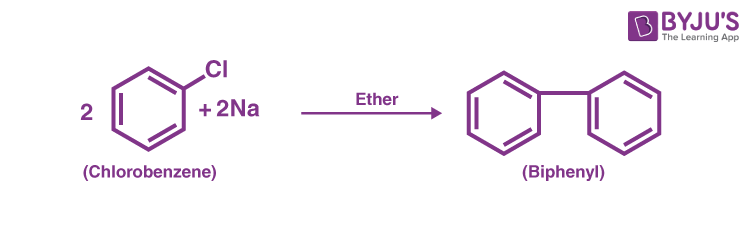(iii) When Methyl chloride is treated with KNO2 nucleophilic substitution takes place due to which, methyl nitrite is formed.

$$\begin{array}{l}C_{2}H_{3}Cl + KNO_{2}\to CH_{3}-O-N=O+KCl\end{array}$$

Test your Knowledge on Important 3 marks questions for cbse class 12 chemistry!